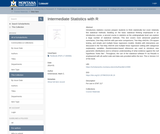# 1 Result

View
Selected filters:
• Montana State UniversityConditional Remix & Share Permitted
CC BY-NC
Rating

Introductory statistics courses prepare students to think statistically but cover relatively few statistical methods. Building on the basic statistical thinking emphasized in an introductory course, a second course in statistics at the undergraduate level can explore a large number of statistical methods. This text covers more advanced graphical summaries, One-Way ANOVA with pair-wise comparisons, Two-Way ANOVA, Chi-square testing, and simple and multiple linear regression models. Models with interactions are discussed in the Two-Way ANOVA and multiple linear regression setting with categorical explanatory variables. Randomization-based inferences are used to introduce new parametric distributions and to enhance understanding of what evidence against the null hypothesis “looks like”. Throughout, the use of the statistical software R via Rstudio is emphasized with all useful code and data sets provided within the text. This is Version 3.0 of the book.

Subject:
Statistics and Probability
Material Type:
Textbook
Provider:
Montana State University
Author:
Mark C. Greenwood